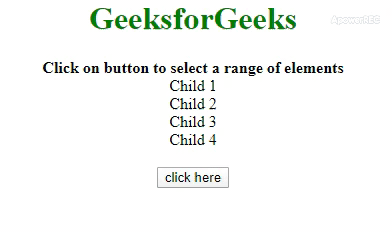# How to Select a Range of Elements using jQuery ?

Given an HTML document with a range of similar elements and the task is to select a range of those elements with the help of JavaScript. There are two approaches that are discussed below with an example.

Approach 1: First, select all elements of class = ‘child’ by jQuery selector then use slice() method to select a range of elements continuously. The background color of the elements has been changed to see the effect.

• Example:

 ` ` `<``html``> ` ` `  `<``head``> ` `    ``<``title``> ` `        ``How to select a range of elements in JQuery ` `    `` ` `    ``<``script` `src``= ` `"https://ajax.googleapis.com/ajax/libs/jquery/3.4.1/jquery.min.js"``> ` `    `` ` `    ``<``style``> ` `        ``h1 { ` `            ``color: green; ` `        ``} ` `         `  `        ``.geeks { ` `            ``color: green; ` `            ``font-size: 24px; ` `            ``font-weight: bold; ` `        ``} ` `    `` ` ` ` ` `  `<``body``> ` `    ``<``center``> ` `        ``<``h1``>  ` `          ``GeeksforGeeks  ` `        `` ` `        ``<``b``>  ` `          ``Click on button to select a range ` `          ``of elements ` `        `` ` `        ``<``div` `class``=``"outer"``> ` `            ``<``div` `class``=``"child"``> ` `              ``Child 1 ` `            ``<``div` `class``=``"child"``> ` `              ``Child 2 ` `            ``<``div` `class``=``"child"``> ` `              ``Child 3 ` `            ``<``div` `class``=``"child"``> ` `              ``Child 4 ` `        `` ` `        ``<``br``> ` `        ``<``button` `onClick``=``"gfg()"``> ` `            ``click here ` `        `` ` `        ``<``p` `id``=``"geeks"``> ` `        `` ` `    `` ` `    ``<``script``> ` `        ``var down = document.getElementById('geeks'); ` `         `  `        ``// Defining childs  ` `        ``var arr = [0, 2, 3]; ` ` `  `        ``function gfg() { ` `            ``var \$el = \$(".outer .child"); ` `            ``for (var i = 0; i < ``arr.length``; i++) { ` `                ``\$el.slice(arr[i], arr[i] + 1) ` `                ``.css("color", "red"); ` `            ``} ` `            ``down.innerHTML` `= ``"Range of elements selected"``; ` `        ``} ` `    `` ` ` ` ` `  ` `

• Output:Approach 2: First select all elements of class = ‘child’ by jQuery selector. There is an array which contains the indexes of the elements to be selected. We are traversing the array and using slice() method to select the particular element of that index. The background color of the elements has been changed to see the effect.

• Example:

 ` ` `<``html``> ` ` `  `<``head``> ` `    ``<``title``> ` `        ``How to select a range of elements in JQuery ` `    `` ` `    ``<``script` `src``= ` `"https://ajax.googleapis.com/ajax/libs/jquery/3.4.1/jquery.min.js"``> ` `    `` ` `    ``<``style``> ` `        ``h1 { ` `            ``color: green; ` `        ``} ` `         `  `        ``.geeks { ` `            ``color: green; ` `            ``font-size: 24px; ` `            ``font-weight: bold; ` `        ``} ` `         `  `    `` ` ` ` ` `  `<``body``> ` `    ``<``center``> ` `        ``<``h1``>  ` `          ``GeeksforGeeks  ` `        `` ` `        ``<``b``>  ` `          ``Click on button to select a range ` `          ``of elements ` `        `` ` `        ``<``div` `class``=``"outer"``> ` `            ``<``div` `class``=``"child"``> ` `              ``Child 1 ` `            ``<``div` `class``=``"child"``> ` `              ``Child 2 ` `            ``<``div` `class``=``"child"``> ` `              ``Child 3 ` `            ``<``div` `class``=``"child"``> ` `              ``Child 4 ` `        `` ` `        ``<``br``> ` `        ``<``button` `onClick``=``"gfg()"``> ` `            ``click here ` `        `` ` `        ``<``p` `id``=``"geeks"``> ` `        `` ` `    `` ` `    ``<``script``> ` `        ``var down = document.getElementById('geeks'); ` `         `  `        ``// Defining childs  ` `        ``var arr = [0, 2, 3]; ` ` `  `        ``function gfg() { ` `            ``var \$el = \$(".outer .child"); ` `            ``for(var i=0; i<``arr.length``; i++) { ` `               ``\$el.slice(arr[i], arr[i]+1) ` `               ``.css("color", "red"); ` `            ``} ` `            ``down.innerHTML` `= ``"Range of elements selected"``; ` `        ``} ` `    `` ` ` ` ` `  ` `

• Output:My Personal Notes arrow_drop_upCheck out this Author's contributed articles.

If you like GeeksforGeeks and would like to contribute, you can also write an article using contribute.geeksforgeeks.org or mail your article to contribute@geeksforgeeks.org. See your article appearing on the GeeksforGeeks main page and help other Geeks.

Please Improve this article if you find anything incorrect by clicking on the "Improve Article" button below.